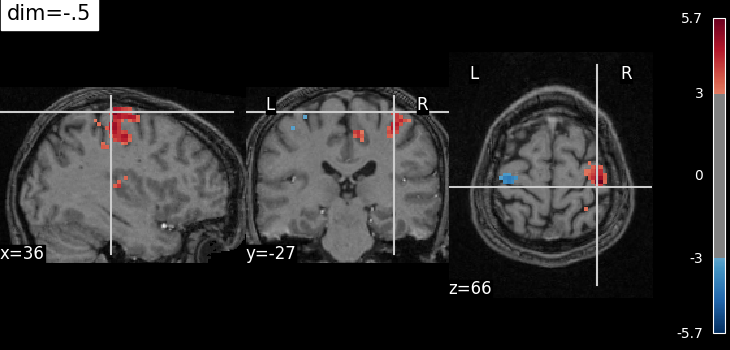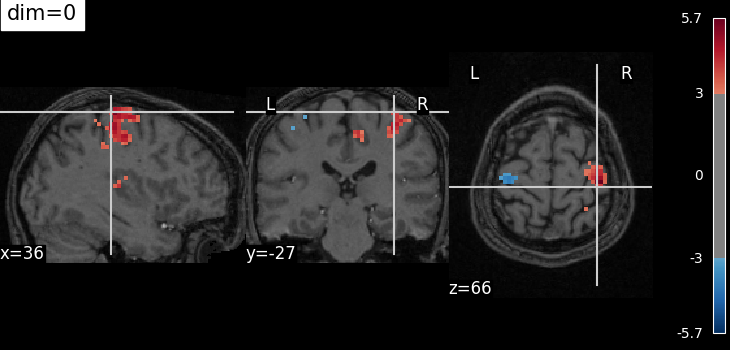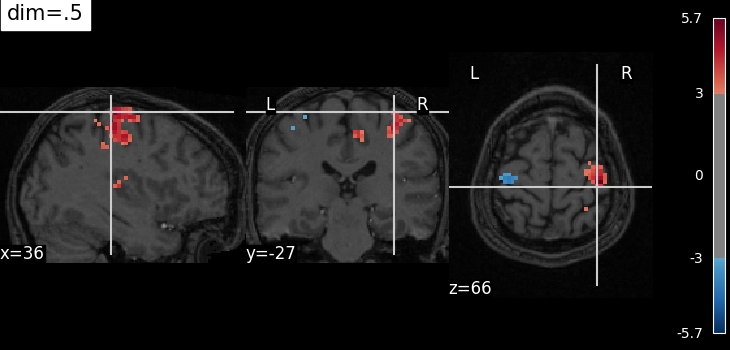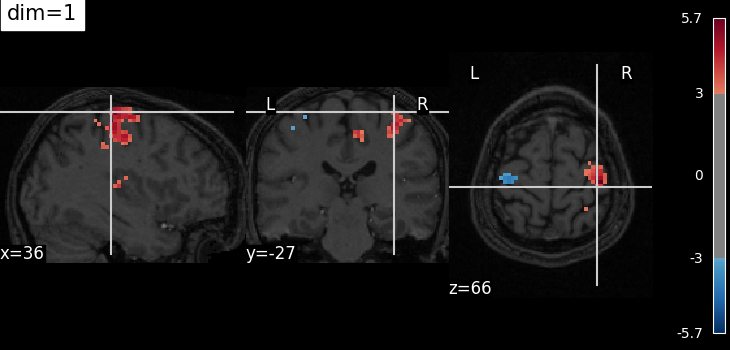# Controlling the contrast of the background when plotting#

The dim argument controls the contrast of the background.

dim modifies the contrast of this image: dim=0 leaves the image unchanged, negative values of dim enhance it, and positive values decrease it (dim the background).

This dim argument may also be useful for the plot_roi function used to display ROIs on top of a background image.

## Retrieve the data: the localizer dataset with contrast maps#

```from nilearn import datasets

# Contrast map of motor task
localizer_tmap_filename = localizer_dataset.tmap
# Subject specific anatomical image
localizer_anat_filename = localizer_dataset.anat
```

## Plotting with enhancement of background image with dim=-.5#

```from nilearn import plotting
plotting.plot_stat_map(localizer_tmap_filename,
bg_img=localizer_anat_filename,
cut_coords=(36, -27, 66),
threshold=3, title="dim=-.5",
dim=-.5)
``````<nilearn.plotting.displays._slicers.OrthoSlicer object at 0x7f1275337340>
```

## Plotting with no change of contrast in background image with dim=0#

```plotting.plot_stat_map(localizer_tmap_filename,
bg_img=localizer_anat_filename,
cut_coords=(36, -27, 66),
threshold=3, title="dim=0",
dim=0)
``````<nilearn.plotting.displays._slicers.OrthoSlicer object at 0x7f12723cba60>
```

## Plotting with decrease of contrast in background image with dim=.5#

```plotting.plot_stat_map(localizer_tmap_filename,
bg_img=localizer_anat_filename,
cut_coords=(36, -27, 66),
threshold=3, title="dim=.5",
dim=.5)
``````<nilearn.plotting.displays._slicers.OrthoSlicer object at 0x7f12753354b0>
```

## Plotting with more decrease in contrast with dim=1#

```plotting.plot_stat_map(localizer_tmap_filename,
bg_img=localizer_anat_filename,
cut_coords=(36, -27, 66),
threshold=3, title="dim=1",
dim=1)

plotting.show()
```Total running time of the script: ( 0 minutes 9.063 seconds)

Estimated memory usage: 9 MB

Gallery generated by Sphinx-Gallery